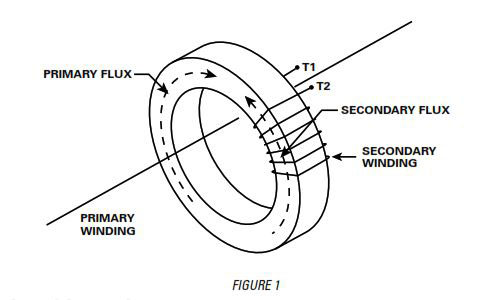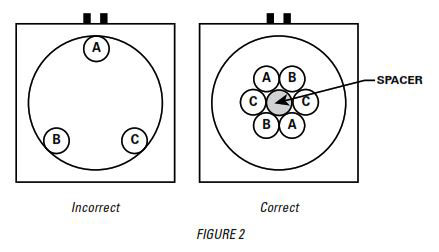• littelfuse

# Current Transformer Application

Littelfuse 10/9/2018
Current Transformer Application

Current Transformers (CTs)

A current transformer is defined as a transformer that produces a current in its secondary circuit that is in proportion to current in its primary circuit.

Although there are other types of CTs, only the window (or ring) type will be discussed here. Window-type CTs get their name from their design that consists of a ring-shaped core. This core is formed by a single length of strip ferromagnetic material tightly wound to form the ring-shaped core.

A CT operates on a principle of flux balance, as shown in Figure 1. If the primary winding is energized with the secondary circuit open-circuited, the transformer becomes an iron-cored inductor. The primary current generates a magnetic flux in the core as shown (flux direction can be determined by the right-hand rule). When the secondary winding is connected to a burden or is short circuited, current flows through the secondary winding creating magnetic flux in the core in opposition to the magnetizing flux created by the primary current. If losses are ignored, the secondary flux balances exactly to the primary flux. This phenomenon is known as Lenz's Law.The secondary lead resistance of CTs cannot be ignored, particularly with low Volt-Amperes (VA) CTs. For example, let's look at an Electronic Overload Relay.

The relay's CT input impedance or burden (ZB) = 0.01 0
The maximum current (1) = 10 A
The CT rating (P) = 5 VA

Now let's solve for the maximum length of #14 AWG leads that will result in a rated accuracy for a 10 A secondary 15 current. Solving for maximum total impedance (ZT):

P = 1^2 ZsubT
ZsubT = P / 1^2 = 5 / 10^2 = 0.05 omega

Solving for the maximum lead resistance (ZsubW):
ZsubT = ZsubW + ZsubB
ZsubW = 0.05 - 0.01 = 0.04 0
If we look up the #14 AWG resistance we find it equals
2.6 ohms / 1000 ft
Therefore, lead length = ZsubW / #14 AWG resistance
Maximum lead length = (0.04 x 1000) / 2.6 = 15.4 ft

CT Installation

A CT should not be operated with its secondary open- circuited. If the secondary is opened when primary current is flowing, the secondary current will attempt to continue to flow so as to maintain the flux balance. As the secondary circuit impedance increases from a low value to a high value the voltage across the secondary winding will rise to the voltage required to maintain current flow. If the secondary voltage reaches the breakdown voltage of the secondary winding, the insulation will fail and the CT will be damaged. Furthermore, this situation presents a personnel shock hazard.

When a ring-type CT is used to monitor a single conductor or multiple conductors, the conductors should be centered in the CT window, as shown below in Figure 2, and should be perpendicular to the CT opening.

In some applications it is difficult or impossible to install the primary conductor through the CT window (example: existing bus bar structure). For these applications a split core CT is sometimes used. Performance of split core CTs may be less than that of solid core CTs.CT characteristics are normally specified at a single frequency such as 50 or 60 Hz. Therefore the question arises: What happens when CTs are used with variable frequency drives (VFDs)? For CTs that are linear to approximately 10x rated primary current at 60 Hz, the VoltsIHertz ratio is approximately constant. That is, for all other conditions held the same at 6 Hz, the CT will be linear to only 1x rated current and at 30 Hz the CT will be linear to 5x rated current. For a standard silicon-steel-core CT, the upper bandwidth frequency is approximately 5 kHz.